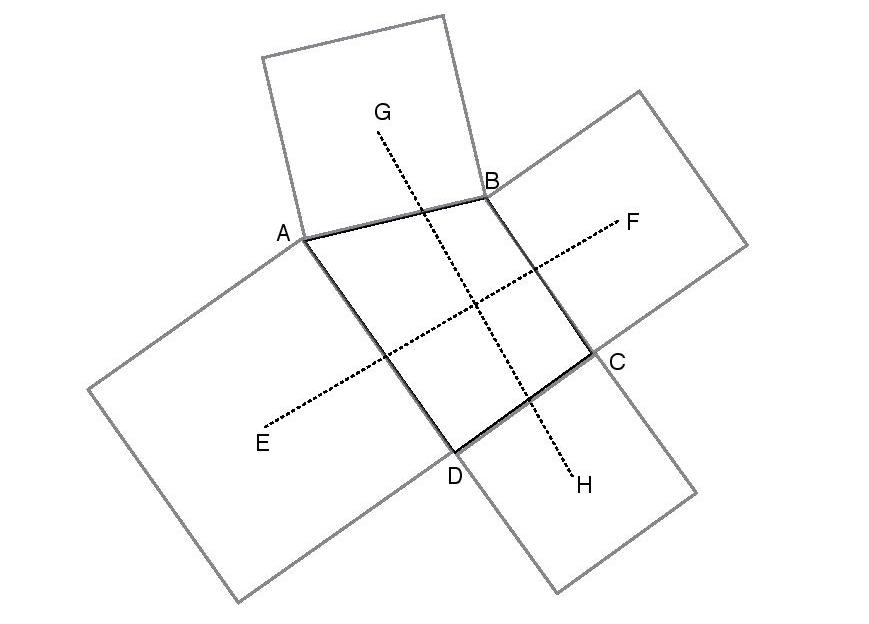# Let's Do Geometry The Complex Way!

Geometry Level 3Consider a quadrilateral $ABCD$ with all distinct sides as shown above. Squares are constructed on each of its sides as shown in the figure above. Suppose $E, F, G$ and $H$ be the centres of these squares.

Let $r=\dfrac{\text{length}(GH)}{\text{length}(EF)}$ and let $0 ^ \circ \leq \theta \leq 90^\circ$ denote the angle (in degrees) between the lines $GH$ and $EF$.

Find the value of $r+\theta$ .

Bonus: For those who know the result already try doing it in a different way(probably by using Complex Numbers!)

×